Name:    MCAS Centripetal Force and Gravity

Multiple Choice
Identify the choice that best completes the statement or answers the question.

1.

One 7.0 kg bowling ball is lifted to a storage shelf 1.0 m above the floor. A second 7.0 kg ball is lifted to a storage shelf 2.0 m above the floor. Which of the following best explains why the measured force of gravity on each ball is nearly identical? (MCAS 2006)
 a. The final potential energy of each ball increased. b. The amount of work required to lift each ball is identical. c. The distance of each ball from Earth’s center of mass is almost identical. d. The gravitational force of each ball on the other cancels out the force of Earth’s gravity.

2.

The Sun’s gravitational attraction causes a comet’s path to curve as shown in the diagram below.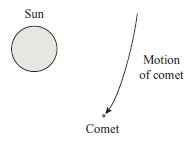Which of the following statements best explains the fact that the Sun does not appear to move due to the comet’s gravitational attraction? (MCAS 2006)
 a. The Sun’s mass is much greater than the comet’s mass. b. The comet is too far away for its gravity to affect the Sun. c. In this gravitational interaction only the comet exerts a pull on the Sun. d. The path of the comet reduces the Sun’s gravity.

3.

The diagram below represents two identical space ships, Earth, a space station, and the Moon.The two ships are launched toward the Moon. Ship 1 is launched from Earth, and ship 2 is launched from the space station. It takes less force to launch ship 2. Which of the following contributes most to the difference in the forces? (MCAS 2007)
 a. Ship 2 has less inertia in space than ship 1 does on Earth. b. Earth exerts less gravitational force on ship 2 than on ship 1. c. Ship 2 must travel a shorter distance than ship 1 before reaching the Moon. d. The Moon exerts more gravitational force on ship 2 than on ship 1.

4.

The illustration below shows an athlete participating in an Olympic hammer throw event.The athlete twirls the hammer (a heavy steel ball attached to a wire) around several times before releasing it. The diagram below shows the path of the ball as it is spun in a circle before its release.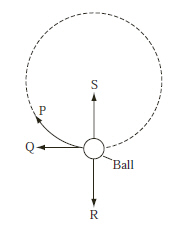Which arrow shows the direction of the centripetal force on the ball before the ball is released? (MCAS 2008)
 a. P b. Q c. R d. S

5.

The distance of the star Vega from Earth is 1.6 million times greater than the distance of the Sun from Earth. Which of the following best describes the gravitational influence of Vega on Earth? (MCAS 2008)
 a. It is roughly equal to that of the Sun. b. Its influence is greater than that of the Sun. c. Its influence is small because of its distance. d. It influences the magnitude of Earth’s mass.

6.

The diagram below shows a ball moving in a circular path.Which of the following would cause the ball to fly off in a straight-line path?
(MCAS 2009)
 a. decreasing the mass of the ball b. changing the velocity of the ball c. increasing the radius of the balls path d. removing the centripetal force on the ball

7.

Tides, such as those along the coast of Massachusetts, are caused by gravitational attractions acting on Earth. Why is the gravitational attraction of the Moon a greater factor in determining tides than the gravitational attraction of the much larger Sun? (MCAS 2004)
 a. Earth is much closer to the Moon than to the Sun. b. The Sun’s gravity is a factor only during the day. c. The Moon’s core has a much greater density than the Sun’s core. d. The Sun’s mass is smaller than the mass of the Moon.

8.

Which of the following statements best describes the force of Earth’s gravity on a rocket moving upward? (MCAS 2010)
 a. The gravitational force is constant for all altitudes. b. The gravitational force is weaker when the rocket is higher. c. The gravitational force is stronger when the rocket is higher. d. The gravitational force is zero when the altitude is greater than 10,000 miles.

9.

Which of the following statements describes a result of Earth’s gravitational pull on the Moon? (MCAS 2010)
 a. The Moon has craters. c. The Moon lacks an atmosphere. b. The Moon orbits around Earth. d. The Moon has less gravity than Earth.

10.

The diagram below shows a comet in an elliptical orbit around a star.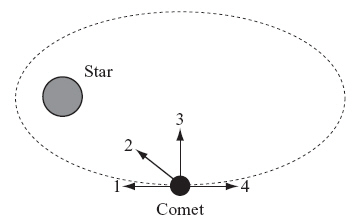Which arrow indicates the direction of the gravitational force the star exerts on the comet when the comet is in the position shown? (MCAS 2011)
 a. 1 b. 2 c. 3 d. 4

11.

A boy has a ball on a string. He swings the ball in a circle above his head. The diagram below is a top view of this situation.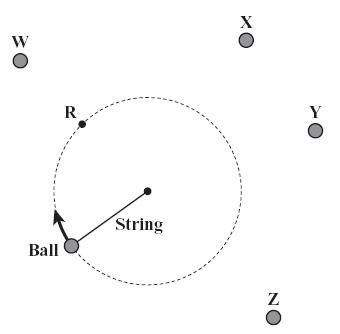If the boy releases the string when the ball is at position R, at which point will the ball land? (MCAS 2011)
 a. point W b. point X c. point Y d. point Z

12.

According to Newton’s law of universal gravitation, in which of the following situations does the gravitational attraction between two bodies always increase? (MCAS 2012)
 a. The masses increase, and the distance between the centers of mass increases. b. The masses increase, and the distance between the centers of mass decreases. c. The masses decrease, and the distance between the centers of mass increases. d. The masses decrease, and the distance between the centers of mass decreases.

13.

A ball is thrown upward at an angle from position P. The diagram below shows the position of the ball at equal time intervals as it moves from position P to position Q.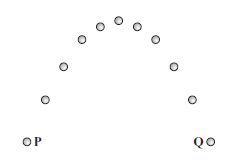Which of the following causes the change in the ball’s velocity as the ball travels from position P to position Q? (MCAS 2012)
 a. decrease in its inertia c. downward force of gravity b. increase in its momentum d. initial acceleration upward

14.

The diagram below shows an object traveling at a constant speed in a circular path.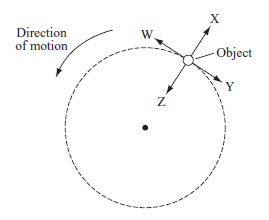Which labeled arrow represents the centripetal force acting on the object? (MCAS 2012)
 a. W b. X c. Y d. Z

15.

A student researches Jupiter and Saturn and records the following information:
• Jupiter is about half the distance to the Sun that Saturn is.
• Jupiter is about three times more massive than Saturn.
Based on this information, which of the following can be concluded about the gravitational forces between these planets and the Sun? (MCAS 2013)
 a. There are no gravitational forces between Jupiter and the Sun or between Saturn and the Sun. b. There are equal gravitational forces between Saturn and the Sun and between Jupiter and the Sun. c. There is a greater gravitational force between Jupiter and the Sun than between Saturn and the Sun. d. There is a greater gravitational force between Saturn and the Sun than between Jupiter and the Sun.

16.

The diagram below shows a ball tied to a string. A student is swinging the ball in a horizontal circle.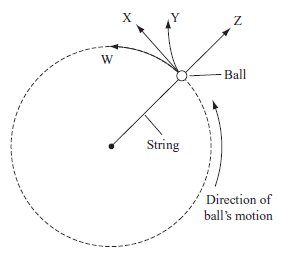If the student releases the string, in which direction will the ball travel? (MCAS 2013)
 a. direction W b. direction X c. direction Y d. direction Z

17.

The Moon was much closer to Earth 4.6 billion years ago. Which of the following statements describes the gravitational interaction of Earth and the Moon 4.6 billion years ago? (MCAS 2014)
 a. The gravitational force of the Moon on Earth was larger than the force is now. b. The gravitational force of Earth on the Moon was the same as the force is now. c. The gravitational force of the Moon on Earth was smaller than the force is now. d. The gravitational force of Earth on the Moon was smaller than the force is now.

18.

A space station moves in a circular orbit around Earth. Which of the following statements describes the centripetal force acting on the space station? (MCAS 2014)
 a. The force is acting in the direction of motion. b. The force is directed toward the space station. c. The force is directed toward the center of Earth. d. The force is acting opposite the direction of motion.

Essay

19.

An attractive force is responsible for keeping satellites in orbit around Earth. The table below gives the masses of seven satellites and the distances between each of these satellites and the center of Earth. (MCAS 2009)

 Satellite Mass (kg) Distance between Satellite and Center of Earth (km) 1 50 7,000 2 100 14,000 3 200 7,000 4 200 14,000 5 400 14,000 6 400 28,000 7 800 28,000

a.       Identify the attractive force that holds satellites in orbit.

b.       Explain why satellite 3 does not experience the same amount of attractive force as satellite 1.

c.       Identify one other satellite that experiences the same amount of attractive force as satellite 4, and explain why satellite 4 and the satellite you identified experience the same attractive force.